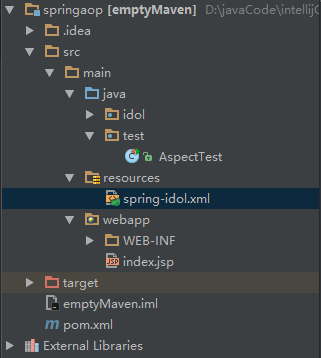+关注继续查看

# 使用 LaTeX 渲染文本

Matplotlib 可以选择使用 LaTeX 来管理所有文本布局。 此选项可用于以下后端：

• Agg
• PS
• PDF

LaTeX 选项通过在rc设置中设置text.usetex:True来激活。 使用 matplotlib 的 LaTeX 支持的文本处理会慢于 matplotlib 的非常强大的 mathtext，但是更灵活，因为可以使用不同的 LaTeX 包（字体包，数学包等）。 结果会十分惊人，特别是当你在图形中使用和主文档相同的字体。

Matplotlib 的 LaTeX 支持需要可用的 LaTeX 安装版本，dvipng（可能包括在你的 LaTeX 安装中）和 Ghostscript（建议使用 GPL Ghostscript 8.60 或更高版本）。 这些外部依赖的可执行文件必须都位于你的PATH中。

font.family        : serif
font.serif         : Times, Palatino, New Century Schoolbook, Bookman, Computer Modern Roman
font.sans-serif    : Helvetica, Avant Garde, Computer Modern Sans serif
font.cursive       : Zapf Chancery
font.monospace     : Courier, Computer Modern Typewriter

text.usetex        : true

from matplotlib import rc
rc('font',**{'family':'sans-serif','sans-serif':['Helvetica']})
## for Palatino and other serif fonts use:
#rc('font',**{'family':'serif','serif':['Palatino']})
rc('text', usetex=True)

"""
Demo of TeX rendering.

You can use TeX to render all of your matplotlib text if the rc
parameter text.usetex is set.  This works currently on the agg and ps
backends, and requires that you have tex and the other dependencies
described at http://matplotlib.org/users/usetex.html
properly installed on your system.  The first time you run a script
you will see a lot of output from tex and associated tools.  The next
time, the run may be silent, as a lot of the information is cached in
~/.tex.cache

"""
import numpy as np
import matplotlib.pyplot as plt

# Example data
t = np.arange(0.0, 1.0 + 0.01, 0.01)
s = np.cos(4 * np.pi * t) + 2

plt.rc('text', usetex=True)
plt.rc('font', family='serif')
plt.plot(t, s)

plt.xlabel(r'\textbf{time} (s)')
plt.ylabel(r'\textit{voltage} (mV)',fontsize=16)
plt.title(r"\TeX\ is Number "
r"$\displaystyle\sum_{n=1}^\infty\frac{-e^{i\pi}}{2^n}$!",
fontsize=16, color='gray')
# Make room for the ridiculously large title.

plt.savefig('tex_demo')
plt.show()# $% & ~ _ ^ \ { }    所以，这些字符会根据rcParam text.usetex标志位而表现不同。 ## 在 TeX 中使用 Unicode 也可以在 LaTeX 文本管理器中使用 unicode 字符串，这里是从tex_unicode_demo.py中获取的示例： # -*- coding: utf-8 -*- """ This demo is tex_demo.py modified to have unicode. See that file for more information. """ from __future__ import unicode_literals import numpy as np import matplotlib matplotlib.rcParams['text.usetex'] = True matplotlib.rcParams['text.latex.unicode'] = True import matplotlib.pyplot as plt plt.figure(1, figsize=(6, 4)) ax = plt.axes([0.1, 0.1, 0.8, 0.7]) t = np.arange(0.0, 1.0 + 0.01, 0.01) s = np.cos(2*2*np.pi*t) + 2 plt.plot(t, s) plt.xlabel(r'\textbf{time (s)}') plt.ylabel('\\textit{Velocity (\u00B0/sec)}', fontsize=16) plt.title(r'\TeX\ is Number$\displaystyle\sum_{n=1}^\infty'
r'\frac{-e^{i\pi}}{2^n}\$!', fontsize=16, color='r')
plt.grid(True)
plt.show()## 可能的问题

• 在 Windows 上，可能需要修改PATH环境变量来包含 latex，dvipng 和 ghostscript 可执行文件的目录。详细信息请参阅环境变量在 Windows 中设置环境变量
• 使用 MiKTeX 与 Computer Modern 字体，如果你得到奇怪的 *Agg 和 PNG 结果，访问MiKTeX/Options并更新你的格式文件。
• 字体在屏幕上看起来糟糕。你可能正在运行 Mac OS，在 mac 上的老版本 dvipng 运行着一些有趣的事情。在你的matplotlibrc文件中设置text.dvipnghack:True
• 在 Ubuntu 和 Gentoo 上，texlive 基本安装不附带 type1cm 包。你可能需要安装一些额外的包，来获得所有与其它 LaTeX 分发版捆绑的特性。
• matplotlib 已经取得了一些进展，所以可以直接使用dvi文件进行文本布局。这允许 LaTeX 用于具有pdfsvg后端的文本布局，以及 *Agg 和 PS 后端。在将来，LaTeX 安装可能是唯一的外部依赖。

## 故障排除

• 尝试删除.matplotlib/tex.cache目录。 如果你不知道.matplotlib在哪里，请参见 matplotlib 配置和缓存目录位置
• 确保 LaTeX，dvipng 和 ghostscript 都正常工作，并存在于你的PATH中。
• 确保你想要做的事情在 LaTeX 文档中可实现，你的 LaTeX 语法是有效的，并且你正在使用原始字符串，如果必要，以避免意外的转义序列。
• 邮件列表上报告的大多数问题已通过升级 Ghostscript 来清除。 如果可能的话，请尝试升级到最新版本，然后向列表报告问题。
• text.latex.preamble rc设置不受官方支持。 此选项提供了大量的灵活性和导致问题的许多方法。 请在向邮件列表报告问题之前禁用此选项。
• 如果仍需要帮助，请参阅获取帮助《Raspberry Pi用户指南》——2.4　使用外部存储设备
1881 0DotNetTextbox使用
1075 060 0Android Material Design控件学习（三）——使用TextInputLayout实现酷市场登录效果
1978 0JSP---JSP中4个容器-pageContext使用

724 0549 0《Apache Velocity用户使用指南》翻译邀请
1080 01078 0RichTextBox使用tab缩进

734 01005 0
apachecn_飞龙
Github：@wizardforcel 简书：@ApacheCN_飞龙 微博：@龙雀 CSDN：@wizardforcel ApacheCN 官网：apachecn.org 机器学习交流群：629470233
444

0

《SaaS模式云原生数据仓库应用场景实践》

《看见新力量：二》电子书